ICSE Class 9 Maths Sample Question Paper 9 with Answers

Section – A
(Attempt all questions from this Section)

Question 1.
(a) Find xy, if x + y = 6 and x – y = 4.
Given: x + y =6,x – y=4.
Now, 4xy =(x+y)2-(x-y)2
= 62 – 42
=20
xy=$$\frac{20}{4}$$=5.(b) Find the mean of first 10 prime numbers.
First lo prime numbers are 2, 3, 5, 7, 11, 13, 17, 19, 23, 29
∴ ∑ =129,n=10
Mean = $$\frac{\sum x}{n}=\frac{129}{10}=12.9$$

(c) If x = acosθ + bsinθ and y = asinθ – bcosθ, prove that x2 + y2 = a2 + b2.Question 2.
(a) Simplify : $$\left(\frac{1}{\sqrt{5}-2}-\frac{1}{\sqrt{5}+2}\right)\left(\frac{1}{2+\sqrt{3}}+\frac{1}{2-\sqrt{3}}\right)$$(b) The area of a trapezium is 540 cm2. If the ratio of parallel sides is 7 : 5 and the distance between them is 18 cm, find the length of parallel sides.
Given : Area of trapezium = 510 cm2
Perpendicular distance between parallel lines = 18 cm
Ratio of parallel sides = 7 : 5
Let the length of parallel sides be 7x and 5x.
Area of trapezium = $$\frac{1}{2}$$ (Sum of parallel sides) x Perpendicular distance7x = 7 x 5 = 35
and 5x = 5 x 5 = 25
Hence, the length of parallel sides are 35 cm and 25 cm.(c) Solve : (3x + 1) (2x + 3) = 3.
(3x + 1) (2x + 3) = 3
6 x 2 + 9x + 2x + 3 – 3 = O
6 x 2 +11x = 0
x (6x + 11) = 0
x=0 or 6x+11=0
x = 0 or x=$$\frac{-11}{6}$$
x = 0 or $$\frac{-11}{6}$$

Question 3.
(a) Express $$\log _{10}\left(\frac{a^{3} c^{2}}{\sqrt{b}}\right)$$
A ladder 13 cm long rests against a vertical wall. If the foot of the ladder is 5 m from the foot of the in terms of log10 a, log10 b and log10 c.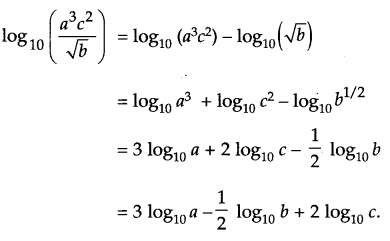(b) A ladder 13 cm long rests against a vertical wall. If the foot of the ladder is 5 m from the foot of the wall, find the distance of the other end of the ladder from the ground.
Let AC be the ladder and BC be the height of the wall.
Then, AC = 13m, AB= 15m, ∠B=90°
∴ By Pythagoras theorem,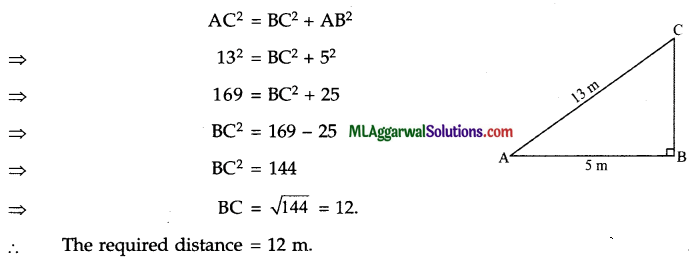(c) Factorize : a4 + b4 – 11a2 b2.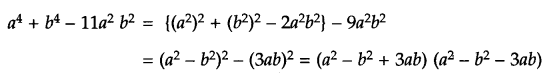Question 4.
(a) Simplify: $$\sqrt{\frac{1}{4}}+(0.04)^{-1 / 4}-(8)^{2 / 3}$$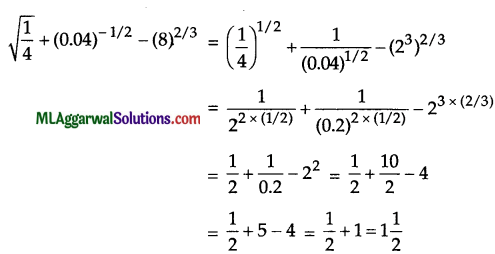(b) In the figure, ABCD is a trapezium in which DA || CB. AB has been produced to E. Find the angles of the trapezium.Given: DA || CB
x + + 100 = 180° (Co-interior angles) … (i)
⇒ x+2y = 170°
Also, x + 25° = y (Corresponding angles) … (ii)
x – y= – 25°
Subtracting equation (ii) from equation (i), we get
3y = 195°
= $$y=\frac{195^{\circ}}{3}=65^{\circ}$$
Putting y = 65° in equation (ii), we get
X – 65° = – 25°
x = 65 – 25°=40°
∠A = x + 25° = 40°+ 25° = 65°
∠B = 180° – y= 180 – 650= 115°
∠C = 2y + 10° =2 x 65° + 10° = 1400
∠D = x = 40°

(c) Calculate the compound interest for the second year on ₹ 8000 when invested for 3 years at 10% p.a.Section – B
(Attempt any four questions from this Section)

Question 5.
(a) Solve for x : 25x – 1 = 52x – 1 – 100.(b) In a ΔABC, ∠A = 80°, ∠B = 40° and bisectors of ∠B and ∠C meet at O. Find ∠BOC.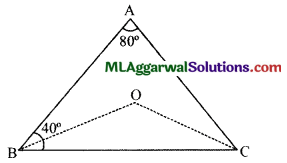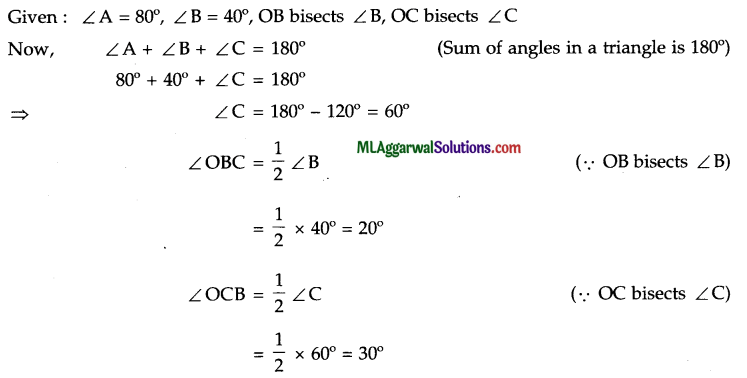(c) Without using tables, evaluate :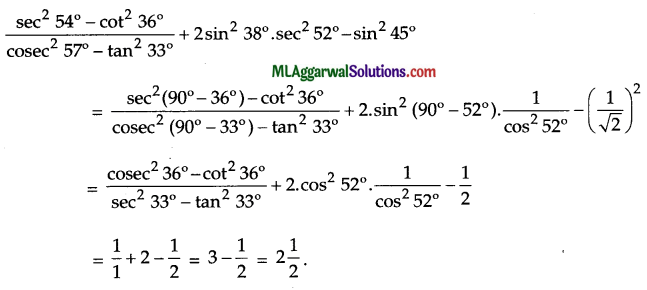Question 6.
(a) If x = 3 + 2√2 , find the value of $$x^{3}-\frac{1}{x^{3}}$$(b) Construct a rectangle each of whose diagonals measures 6 cm and the diagonals intersect at an angle of 45°.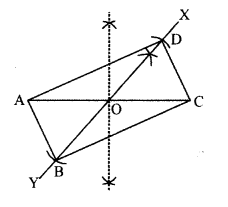Given: Each diagonal = 6 cm, diagonals intersect at 45°.
Steps of construction:
(1) Draw diagonal AC = 6 cm.
(2) Bisect AC at O
(3) At O, draw ∠COX = 45° and extend XO to Y.
(4) From O, cut-off XY at D and B such that OD = OB = 3 cm,
i.e., BD = 6 cm.
(5) Join A, B, C, D to get the required rectangle ABCD.(c) KM is a straight line of 13 units. If K has the coordinates (2, 5) and M has the coordinates (x, – 7), find the possible values of x.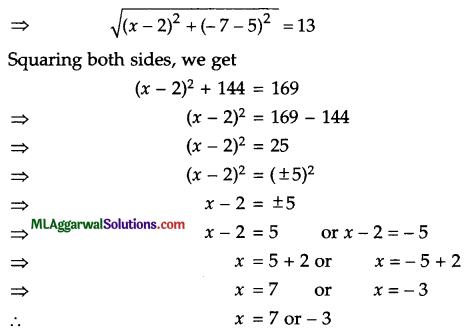Question 7.
(a) If $$x=\frac{\sqrt{7}+1}{\sqrt{7}-1} \text { and } y=\frac{\sqrt{7}-1}{\sqrt{7}+1}$$find the value of $$\frac{x^{2}+x y+y^{2}}{x^{2}-x y+y^{2}}$$(b) In ΔABC, AC = 3 cm. If M is the mid-point of AB and a straight line through M parallel to AC cuts BC at N, what is the length of MN ?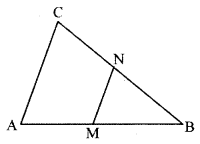Given : AC = 3 cm, M is the mid-point of AB, MN || AC.
Since, M the mid-point of AB and MN || AC
Therefore, by mid-point theorem, we have
MN = $$\frac{1}{2}$$AC= $$\frac{1}{2}$$ x 3cm = 1.5cm(c) If θ is an acute angle and tan θ = $$\frac{5}{12}$$, find the value of cosθ + cot θ.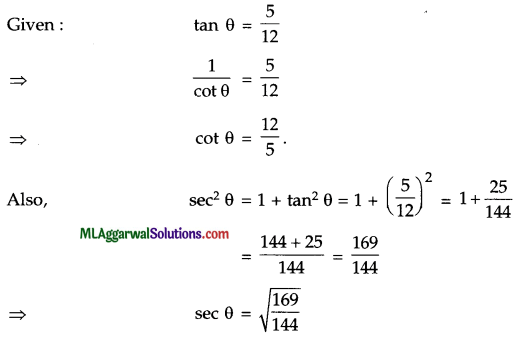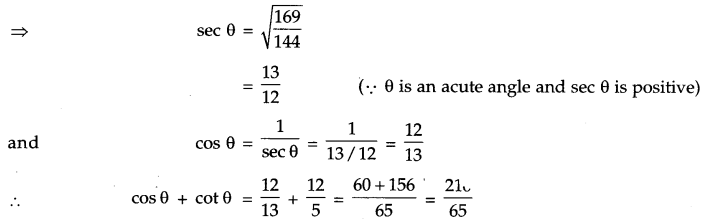Question 8.
(a) Factorize : 1 – 2ab – (a2 + b2)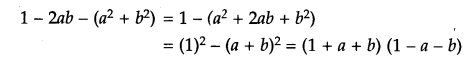(b) In the given figure, AOC is a diameter of a circle with centre O and arc A×B = $$\frac{1}{2}$$ arc BYC. Find ∠BOC.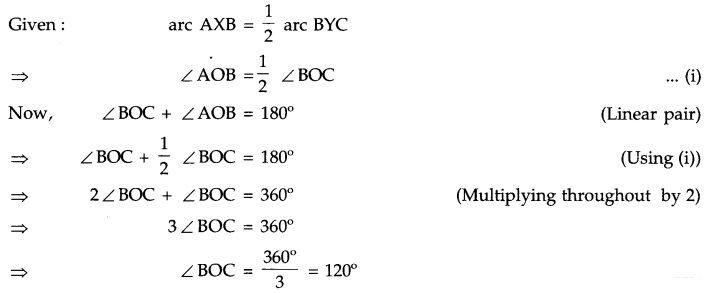(c) In a class of 90 students, the marks obtained in a weekly test were as under.Construct a combined histogram and frequency polygon.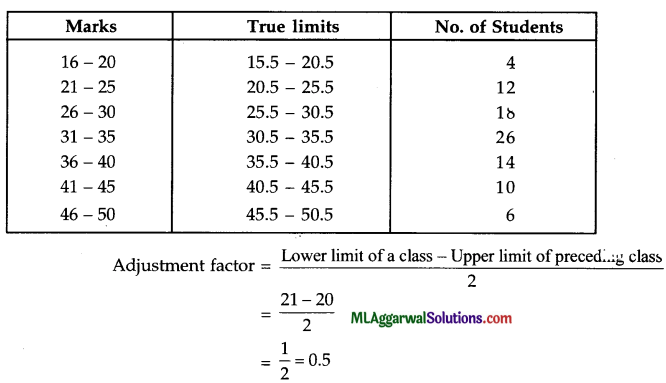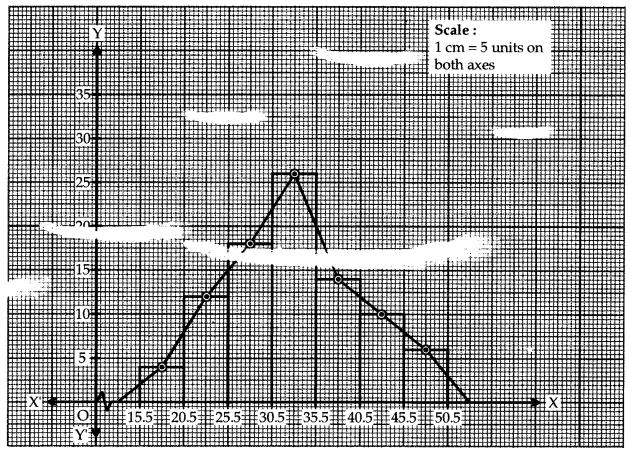Question 9.
(a) Divide ₹ 1,95,150 between A and B so that the amount that A receives in 2 years is the same as that of B receives in 4 years. The interest is compounded annually at the rate of 4% p.a.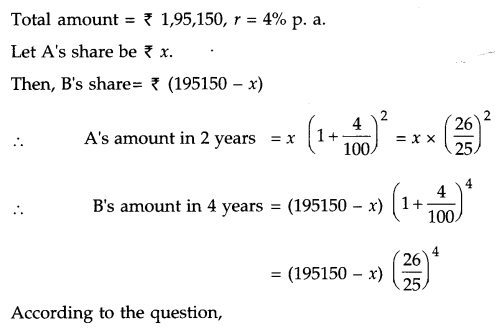A’s share = 1,01,400.
and B’s share = (1,95,150 – 1,01,400) = ₹ 93,750.(b) Solve simultaneously : $$\frac{3}{x+y}+\frac{2}{x-y}=3 ; \frac{2}{x+y}+\frac{3}{x-y}=\frac{11}{3}$$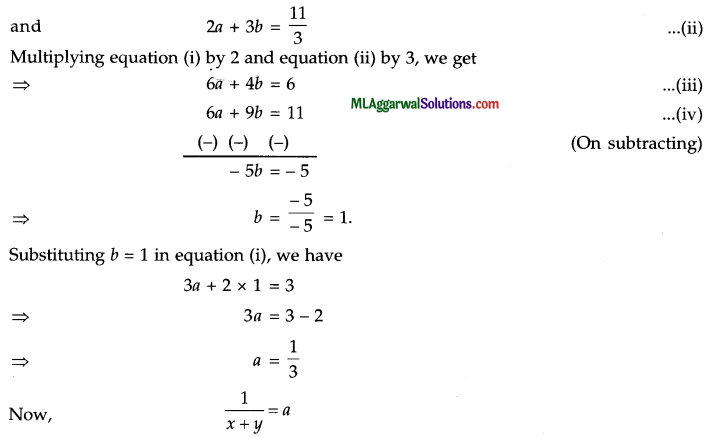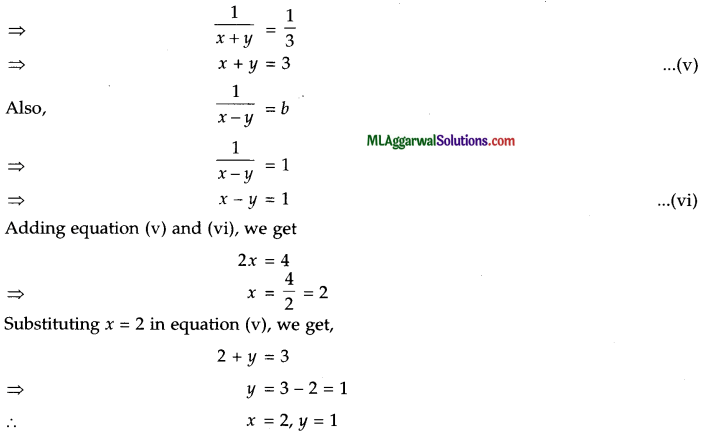(c) If a = cz, b = ax and c = by, prove that xyz = 1.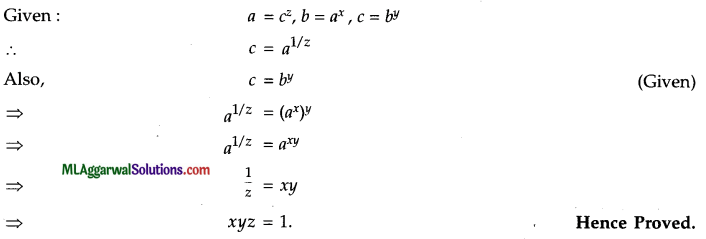Question 10.
(a) If sin (A + B) = 1 and cos (A – B) = $$\frac{\sqrt{3}}{2}$$, 0° < A + B ≤ 90°, A > B, then find A and B.
sin (A + B) = 1 = sin 90°
A + B = 90°
cos (A – B) =$$\frac{\sqrt{3}}{2}$$ = cos 30°
A – B = 30°
Adding (i) and (ii), we get
A + B + A – B = 90° + 30°
⇒ 2A = 120°
A = $$\frac{120^{\circ}}{2}$$ = 60°
Putting A= 60° in (i), we get
60° + B = 90°
⇒ B = 90° – 60° = 30°
∴ A = 60°, B = 30°(b) The sum of the digits of a two-digit number is 5. The digit obtained by increasing the digit in ten’s place by unity is one-eighth of the number. Find the number.Let the digit at ten’s place be  and that at unit’s place be y.
∴ The number = 10x + y
By 1st condition,
x + y = 5
By 2nd condition,
x + 1 = $$\frac{1}{8}$$ (10x + y)
⇒ 8 (x + 1) = 10x + y
⇒ 8x + 8 = 10x + y
⇒ 2x + y = 8
Subtracting (i) from (ii), we get
x = 3.
Putting x = 3 in (i), we get
3 + y = 5
⇒ y = 5 – 3 = 2
The required number = 10x + y = 10 x 3 + 2 = 32(c) Given, log10 x = a and log10 y =
(i) Write down 10a-1 in terms of x
(ii) Write down 102b in terms of y.
(iii) If log10 P = 2a-b, express P in terms of x and y.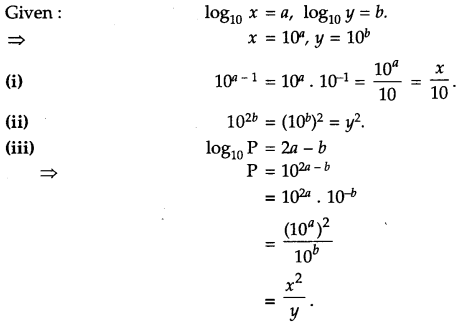Question 11.
(a) Draw the graph of the equations 2x – 3y = 7 and x + 6y = 11 and find their solutions.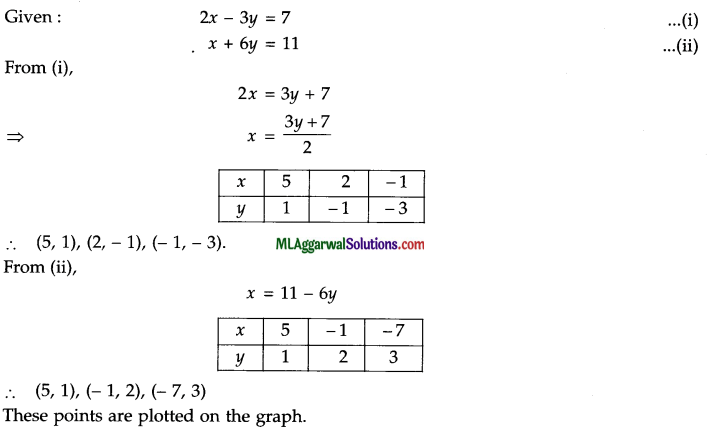The two lines intersect each other at the point (5, 1).
∴ x = 5, y = 1(b) In the figure, area of ΔABD = 24 sq. units. If AB = 8 units, find the height of ΔABC.
Given : Area of A ABD = 24 sq. units, AB = 8 units, DC || AB.
Area of ΔABC = Area of ΔABD
(∵ They are on same base and between same parallels)
= 24 sq. units.
⇒ $$\frac{1}{2}$$ x AB x Height of ΔABC = 24
⇒ $$\frac{1}{2}$$ x 8 x Height of ΔABC = 24
⇒ Height of Δ ABC = $$\frac{24}{4}$$ = 6 units.

(c) If x – y = 8 and xy = 20, evaluate : (i) x + y (ii) x2 – y2.